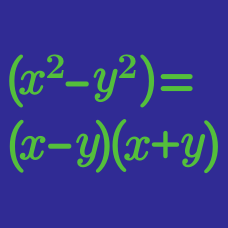Algebra

# Polynomial Factoring

#### Challenge Quizzes

If $a$, $b$, $c$ and $d$ are positive numbers that satisfy $x^4-40x^2+4 = (x^2+ax-b)(x^2-cx-d),$ what is the value of $a+b+c+d$?

If $a$ and $b$ are positive numbers such that $x^3+2x^2y-9x-18y=(x+a)(x-b)(x+cy),$ what is the value of $a+b+c$?

If $b$ and $c$ are positive numbers such that $(x^2-1)(x^2+8x+7)=(x+a)^2(x+b)(x-c),$ what is the value of $a+b+c$?

If $a$ and $b$ are positive integers such that \begin{aligned} & x^2 y^2-9x^2z^2-10xy^2+90xz^2+25y^2-225z^2\\ & =(x-a)^2(y-bz)(y+bz), \end{aligned} what is the value of $a+b$?

A polynomial $x^4-226x^2+225$ can be factorized as $(x-a)(x-b)(x-c)(x-d).$ If the four constants satisfy $a < b < c < d$, what is the value of $bc-ad$?

×